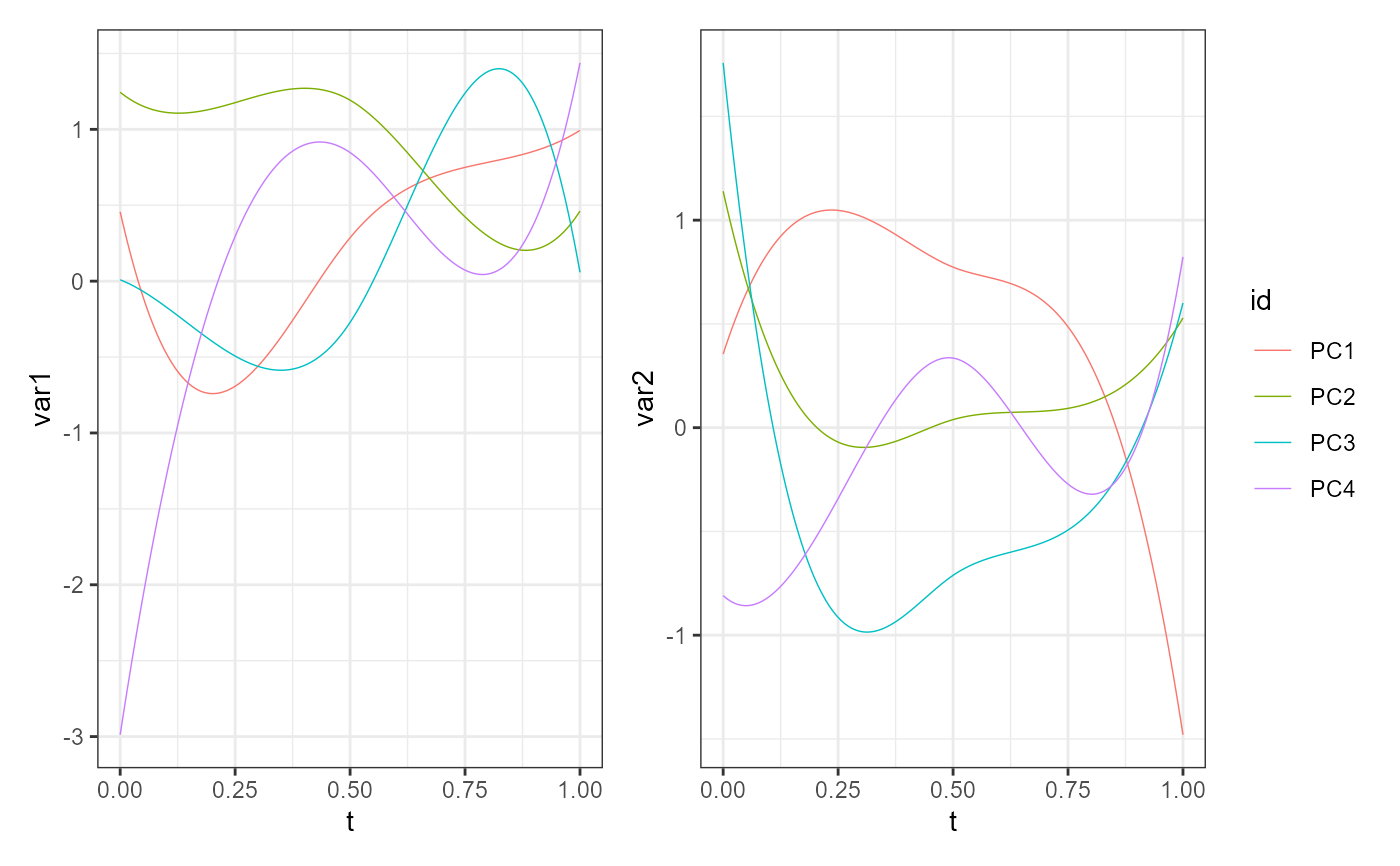Plot the harmonics of a pca_mfd object

plot_pca_mfd(pca, harm = 0, scaled = FALSE)

## Arguments

pca

A fitted multivariate functional principal component analysis (MFPCA) object of class pca_mfd.

harm

A vector of integers with the harmonics to plot. If 0, all harmonics are plotted. Default is 0.

scaled

If TRUE, eigenfunctions are multiplied by the square root of the corresponding eigenvalues, if FALSE the are not scaled and the all have unit norm. Default is FALSE

## Value

A ggplot of the harmonics/multivariate functional principal components contained in the object pca.

## Examples

library(funcharts)
mfdobj <- data_sim_mfd()
pca_obj <- pca_mfd(mfdobj)
plot_pca_mfd(pca_obj)